[BACK]Computer Modeling in Engineering & SciencesDOI: 10.32604/cmes.2021.014407

ARTICLE

A New Modified Inverse Lomax Distribution: Properties, Estimation and Applications to Engineering and Medical Data

Department of Statistics, Faculty of Science, King Abdulaziz University, Jeddah, 21589, Saudi Arabia
*Corresponding Author: Abdullah M. Almarashi. Email: aalmarashi@kau.edu.sa
Received: 24 September 2020; Accepted: 09 February 2021

Abstract: In this paper, a modified form of the traditional inverse Lomax distribution is proposed and its characteristics are studied. The new distribution which called modified logarithmic transformed inverse Lomax distribution is generated by adding a new shape parameter based on logarithmic transformed method. It contains two shape and one scale parameters and has different shapes of probability density and hazard rate functions. The new shape parameter increases the flexibility of the statistical properties of the traditional inverse Lomax distribution including mean, variance, skewness and kurtosis. The moments, entropies, order statistics and other properties are discussed. Six methods of estimation are considered to estimate the distribution parameters. To compare the performance of the different estimators, a simulation study is performed. To show the flexibility and applicability of the proposed distribution two real data sets to engineering and medical fields are analyzed. The simulation results and real data analysis showed that the Anderson-Darling estimates have the smallest mean square errors among all other estimates. Also, the analysis of the real data sets showed that the traditional inverse Lomax distribution and some of its generalizations have shortcomings in modeling engineering and medical data. Our proposed distribution overcomes this shortage and provides a good fit which makes it a suitable choice to model such data sets.

Keywords: Inverse lomax distribution; logarithmic transformed method; order statistics; maximum likelihood estimation; maximum product of spacing; manuscript; preparation; typeset; format

1  Introduction

Inverse Lomax (IL) distribution is a very important lifetime distribution which can be used as a good alternative to the well known distributions such as gamma, inverse Weibull, Weibull and Lomax distributions. It can be considered as a member of generalized beta family of distributions. It has different applications in modelling various types of data including economics and actuarial sciences data because its hazard rate can be decreasing and upside down bathtub shaped. The random variable X is said to have an IL distribution if its probability density function (PDF) is

h(x;α,θ)=αθx-2(1+θx)-(α+1),x>0,α,θ>0.(1)

The cumulative distribution function (CDF) of (1) is

H(x;α,θ)=(1+θx)-α,(2)

where α is the shape parameter and θ is the scale parameter. For more details about the inverse distributions one can refers to . The traditional IL distribution does not give a good fit for modeling many real life data such as in reliability and biological studies. Recently, many generalizations of IL distribution have been developed. Hassan et al.  introduced Weibull IL distribution and studied some of its statistical properties. Maxwell et al.  proposed the Marshall-Olkin IL distribution by adding a new shape parameter for more flexibility. ZeinEldin et al.  used the alpha power method to add a new shape parameter to the IL distribution and proposed a new distribution which called alpha power IL (APIL) distribution. In spite of these generalizations, still a new generalization of IL is needed because these distributions are not able to model various types of data.

The main objective of this paper is to propose a new form of the IL distribution by adding a new shape parameter to the CDF in (2) using the same approach of . They introduced a new method to add an extra shape parameter to an existing distributions which called the logarithmic transformed (LT) method. The LT method has the following CDF and PDF

F(x;λ)=1-log[1-(1-λ)H̄(x)]log(λ)(3)

and

f(x;λ)=(λ-1)h(x)log(λ)[1-(1-λ)H̄(x)].(4)

respectively, with λ>0, λ1. By taking H̄(x)=1-H(x) in (3) of the IL distribution CDF in (2) we obtain a modified logarithmic transformed IL (MLTIL) distribution. Many researchers used the LT method to propose a new continuous distributions by taking the baseline H(x) of any known distribution. [9,10] introduced a generalizations for modified Weibull extension and generalized exponential distributions, respectively.  introduced a logarithmic inverse Lindley distribution by taking the H(x) in (3) of the inverse Lindley distribution. Also, the logarithm transformed Fréchet distribution by . The overall motivations to propose the MLTIL distribution are

1.    To develop different shapes for the PDF and hazard rate function.

2.    To increase the flexibility of the traditional IL distribution in modelling different phenomenons.

3.    To model skewed data which can not be modeled by other traditional models.

4.    To increase the flexibility of the traditional IL distribution properties like mean, variance, skewness and kurtosis.

5.    Two applications showed that the MLTIL distribution provides a better fit than the traditional IL distribution and some of its generalizations.

6.    Another motivation to this article is to use six classical estimation methods to estimate the parameters in order to recommend which method provide the best estimates based on mean square error criteria and via a simulation study.

The hazard rate function of the MLTIL distribution can has decreasing or upside-down shapes depending on its shape parameters which makes the distribution is quite effectively in modelling lifetime data. It can be used as an alternative to IL and inverse Weibull distributions. Simulation results reveal that the Anderson-Darling (AD) estimators perform better than other estimators in terms of minimum mean-squared errors. Finally, the analysis of engineering and medical data sets show the ability of the MLTIL distribution to provide a better fit than some other competitive models. The rest of the paper is organized as follows: In the next Section we describe the MLTIL distribution and a mixture representation of its density. Some of its statistical properties are discussed in Section 3. Six classical estimation methods are considered in Section 4. A simulation study is conducted in Section 5. Two applications are considered in Section 6. In Section 7, the paper is concluded.

2  Model Description

In this section we introduce the MLTIL distribution. Let the random variable X follows the IL distribution with PDF and CDF, respectively, given by (1), (2), then from (1)(3) the PDF of the MLTIL distribution is given by

f(x;λ,α,θ)=(λ-1)αθx-2(1+θx)-(α+1) log(λ)[λ-(λ-1)(1+θx)-α],x>0,λ,α,θ>0,λ1,(5)

and its CDF is

F(x;λ,α,θ)=1-log[λ-(λ-1)(1+θx)-α] log(λ).(6)

The survival function (SF) is given by

S(x;λ,α,θ)=log[λ-(λ-1)(1+θx)-α] log(λ)(7)

and the hazard rate functions is

h(x;λ,α,θ)=(λ-1)αθx-2(1+θx)-(α+1)[λ-(λ-1)(1+θx)-α] log[λ-(λ-1)(1+θx)-α].(8)

For some selected values of the parameters λ, α and θ, Fig. 1 indicates the various shapes of the PDF of the MLTIL distribution, while Fig. 2 shows the different shapes of the hazard rate function. These Figure indicate the flexibility of the MLTIL distribution to model right skewed data as well as the data with decreasing and upside down bathtub shaped.Figure 1: Plots of the MLTIL density for different values of λ, α and θFigure 2: Plots of the MLTIL hazard rate function for different values of λ, α and θ

Now, we can obtain an useful representation for the PDF and CDF of the MLTIL distribution. Using the series representation in the form

(1-q)n-1=j=0(-1)j(n-1j)qj,if|q|<1,n>0positive real non-integer.(9)

Applying (9) for the PDF in (1), we can obtain

f(x)=k=0ϑkh(x;α(k+1),θ),(10)

where h(x;α(k+1),θ) denotes the PDF of the IL distribution with scale parameter θ and shape parameter α(k+1) and

ϑk=λ(k+1)log(λ)(λ-1λ)k+1.

Different structural properties of the MLTIL distribution can be determined using this representation. By integrating (10), the CDF of X is given by

F(x)=k=0ϑkH(x;α(k+1),θ),(11)

where H(.) is the CDF of the IL distribution with scale and shape parameters θ and shape parameter α(k+1), respectively.

3  Properties of the MLTIL Distribution

In this section some statistical properties of the MLTIL distribution are obtained including quintile function, moments, incomplete moments, conditional moments and entropies.

3.1 Quantile Function

For the MLTIL distribution the quantile function, say x = QMLTIL(q), can be obtained by inverting (6) as

F-1(q)=QMLTIL(q)=θ(λ-1λ(1-λ-q))1α-1.(12)

We can easily generate X by taking q as a uniform random variable in (0,1). Based on (12), one can compute the measures of skewness (Sk) and kurtosis (Ku) as follows:

Sk=Q(34)+Q(14)-2Q(12)Q(34)-Q(14) and Ku=Q(78)-Q(58)+Q(38)-Q(18)Q(68)-Q(28), respectively, where Q() refers to the quantile function.

3.2 Moments

The nth moment of MLTIL distribution can be derives as μn=E(Xn)=-xnf(x)dx=k=0ϑk0xnh(x;α(k+1),θ)dx=k=0ϑkα(k+1)θnB(1-n,α(k+1)+n),n=1,2,3, where B(a,b) is the beta function, Γ(0)=-γ and Γ(-n)=(-1)nn!ϕ(n)-(-1)nn!γ for n=1,2,3,,γ denotes Euler’s constant and ϕ(n)=j=1n1j. For more details see . Similarly, for the MLTIL distribution we can obtain the nth inverse moment as follows: E(1Xn)=k=0ϑkB(1+n,α(k+1)-n)θn.

In particular,

μ1=E(X)=k=0ϑkα(k+1)θΓ(0), where γ denotes Euler’s constant. μ2=E(X2)=k=0ϑkα(k+1)(α(k+1)+1)θ2Γ(-1) and var(X)=μ2-(μ1)2.

The rth central moment μr of X is derived as

μn=E(X-μ1)n=k=0n(-1)r-k(nk)μn-k(μ1)k.

The Sk and Ku measures can be computed using the following expressions:

Sk=μ33μ2μ+2μ3(μ2μ2)32,Ku=μ44μ3μ+6μ2μ23μ4(μ2μ2)2.

The following propositions are a description of three different types of moments such as incomplete moments, moment generating function (mgf) and conditional moment.

prop 3.1. If X~MLTIL, then the incomplete moments of X is given as follows:

IX(t)=k=0ϑktxnh(x;α(k+1),θ)dx            =k=0ϑk0txn(αθ(k+1)x2(1+θx)α(k+1)1)dx            =αθk=0ϑk(k+1)0txα(k+1)+n1(θ+x)α(k+1)1dx            =k=0ϑkα(k+1)tn(tθ)α(k+1)α(k+1)+nF2:1(α(k+1)+1;α(k+1)+n;α(k+1)+n+1;tθ),(13)

The first incomplete moment, I1(t), follows from Eq. (13) with n = 1.

prop 3.2. If X~MLTIL, then the mgf of X is

MX(t)=j=0tjj!E(Xj)=j=0k=0ϑk(θt)jj!α(k+1)B(1-j,α(k+1)+j).

prop 3.3. If X~MLTIL, then the characteristic generating function (cgf) of X is

MX(t)=j=0(it)jj!E(Xj)=j=0k=0ϑk(θit)jj!α(k+1)B(1-j,α(k+1)+j).

prop 3.4. If X~MLTIL, then the conditional moment of X is

CX(t)=E(E(XnX>x)=ψn(x)1F(x), where ψn(x)=k=0ϖkxynh(x;α(k+1),θ)dy=k=0ϑkα(k+1)θ(-θ)n-1B-θx(1-n,-α(k+1)), where, Bz(a,b)=0zya-1(1-y)b-1dy is the incomplete beta function.

3.3 Entropies

Entropy has been used in areas like in physics (sparse kernel density estimation), medicin (molecular imaging of tumors) and engineering (measure the randomness of systems). The entropy is a measure of variation of the uncertainty. The Rényi entropy (RE) of order ρ is defined as

IR(ρ)=11-ρlog(fρ(x)dx).(14)

For the MLTIL distribution in (1), we can write

IR(ρ)=11ρlog{ ((λ1)αθlog(λ))ρ0(x2(1+θx)(α+1)λ(λ1)(1+θx)α)ρdx },ρ0, ρ1           =11ρlog{ ((λ1)αλθlog(λ))ρk=0((λ1)λ)kΓ(ρ+k)k!B(2ρ1,α(k+ρ)ρ+1). }.

The ρ-entropy, Hρ-R(x), can be written as

Hρ-R(ρ)=11-ρlog(1-0fρ(x)dx).(15)

Shannon’s entropy (SE) is defined as E[-logf(X)] and it is a special case of (15) for ρff1. Limiting ρff1 in (15) and using L´Hospital´s rule, one obtains

E[logf(X)]=E[ log((λ1)αθx2(1+θx)(α+1)log(λ)[ λ(λ1)(1+θx)α ]) ]      =log[ (λ1)αθlog(λ) ]E[ log(x2(1+θx)(α+1)λ(λ1)(1+θx)α) ]      =log[ log(λ)αθ(λ1) ]+2E(log(x))+(α+1)E(log(1+θx))      +E(log[ λ(λ1)(1+θx)α ]).

3.4 Distribution of Order Statistics

Let X(1)<X(2),,<X(n) be the order statistics of a random sample of size n taken from the MLTIL distribution. In this case, we can write the PDF of the sth order statistic as follows

fX(s)(x)=f(x)B(s,n-s+1)k=0n-s(-1)k(n-sk)Fk+s-1(x),(16)

where B(., .) is the beta function. Using (1), (6) and a power series expansion, we obtain

f(x)Fk+s-1(x)=αθ(λ-1)(log(λ))k+sx-2(1+θx)-(α+1)λ-(λ-1)(1+θx)-α[log(λλ-(λ-1)(1+θx)-α)]k+s-1.(17)

From (17) and (16), we can write

fX(s)(x)=αθ(λ1)(B(s,ns+1)k=0ns(1)k(log(λ))k+s(nsk)x2(1+θx)(α+1)(λ(λ1)(1+θx)α)                   ×[ log(λλ(λ1)(1+θx)α) ]k+s1.(18)

Particularly, we can obtain the PDF of the first and last order statistics from (18), respectively, as

fX(1)(x)=αθ(λ1)nk=0n1(1)k(log(λ))k+1(n1[3pt]k)x2(1+θx)(α+1)(λ(λ1)(1+θx)α)   ×[ log(λλ(λ1)(1+θx)α) ]k,(19)

and

fX(n)(x)=αθ(λ-1)n(log(λ))nx-2(1+θx)-(α+1)(λ-(λ-1)(1+θx)-α)[log(λλ-(λ-1)(1+θx)-α)]n-1.(20)

3.5 Probability Weighted Moments

The (u,v)th probability weighted moments of the random variable X is defined by

ρu,v=E{XuF(X)v}=-xuf(x)Fv(X)dx.(21)

Based on Eq. (21), we can obtain the probability weighted moments of the MLTIL distribution as

ρu,v=αθ(λ-1)ρ(log(λ))v+1,(22)

where

ρ=0xu-2(1+θx)-(α+1)λ-(λ-1)(1+θx)-α[log(λλ-(λ-1)(1+θx)-α)]vdx.(23)

3.6 Residual Life and Reversed Failure Rate Function

The nth moment of the residual life (RL) of the random variable X is defined as follows

Rn(t)=E((X-t)nX>t)=11-F(t)t(x-t)nf(x)dx,n1

Based on Eq. (1) and applying the binomial expansion of (xt)n, we have

Rn(t)=11F(t)m=0n(t)nm(nm)txnf(x)dx            =αθ(λ1)λm=0nk=0(t)nm(θ)n1(n[3pt]m)(λ1λ)kBθt(1n,(k+1)α)log(λ(λ1)(1+θx)α).

The nth moment of the reversed RL can be derived using the general formula

mn(t)=E((t-X)nXt)=1F(t)0t(t-x)nf(x)dx,n1.

Based on Eq. (1) and applying the binomial expansion of (tx)n, we can write

mn(t)=αθ(λ-1)λlog(θ)F(t)m=0n(-t)n-m(nm)0txnf(x)dx=α(λ-1)λlog(θ)F(t)m=0nk=0(-1)n-m(nm)(λ-1λ)kt2n-m+α(k+1)θα(k+1)(n+α(k+1))×F2:1(a(k+1)+1;n+a(k+1);n+a(k+1)+1;-tθ).

3.7 Stress-Strength Model

Let Y1~MLTIL(λ1,α1,θ1) and Y2~MLTIL(λ2,α2,θ2). If Y1 represents stress and Y2 represents strength, then the stress-strength parameter, denoted by R, for the MLTIL distribution is given by

R=P(Y2>Y1)=0F1(y)dF2(y)=10F¯1(y)dF2(y)=1(λ21)α2θ2log(λ1)log(λ2)0y2(1+θ2y)(α2+1)log[ λ1(λ11)(1+ θ 1x)α1 ]λ2(λ21)(1+θ2x)α2dy=1(λ21)α2θ2log(λ1)log(λ2)0y2(1+θ2y)(α2+1)[ log(λ1)+log(1(1λ1)(1+ θ 1 x)α1) ]λ2(λ21)(1+θ2x)α2dy=1(λ21)α2θ2log(λ2)0y2(1+θ2y)(α2+1)λ2(λ21)(1+θ2x)α2dy(λ21)α2θ2log(λ1)log(λ2)0y2(1+θ2y)(α2+1)log(1(1λ1)(1+ θ 1x)α1)λ2(λ21)(1+θ2x)α2dy.

Using series expansion in the last equation, we obtain

R=1(λ21)α2θ2log(λ2)m=0(λ21λ2)m0y2(1+θ2y)α2(m+1)1dy(λ21)α2θ2log(λ1)log(λ2)×m=0k=0(1)k1k(λ21λ2)m(1λ1λ1)k0y2(1+θ1y)α1k(1+θ2y)α2(m+1)1dy=1(λ21)α2θ2log(λ2)m=0(λ21λ2)m1λ2θ2(m+1)(λ21)α2θ2log(λ1)log(λ2)m=0k=0j=0(1)k1(θ1)jk×(λ21λ2)m(1λ1λ1)k(α1k+j1j)0y2j(1+θ2y)α2(m+1)1dy=1(λ21)α2θ2log(λ2)m=0(λ21λ2)m1λ2θ2(m+1)(λ21)α2log(λ1)log(λ2)m=0k=0j=0(1)k1(θ1θ2)jk×(λ21λ2)m(1λ1λ1)k(α1k+j1j)B(j+1,α2(m+1)j).

4  Estimation

In this section, six estimation methods are considered to estimate the MLIIL distribution parameters.

4.1 Maximum Likelihood Estimation

Using a random sample of size m taken from the MLTIL distribution, then based on (5) the log-likelihood function can be written as

E1(ϖ)=mlog((λ-1)αθlog(λ))-2j=1m log(xj)-(α+1)j=1m log(1+θxj)-j=1m log[λ-(λ-1)(1+θxj)-α],(24)

where ϖ=(λ,α,θ)T. The maximum likelihood estimates (MLEs) of λ, α, and θ, denoted by λ^ML, α^ML, and θ^ML, are the solution of the following three equations

E1(ϖ)λ=mλ-1-mλlog(λ)-j=1m1-(1+θxj)-αλ-(λ-1)(1+θxj)-α=0, E1(ϖ)α=mα-j=1m log(1+θxj)-j=1m(λ-1)(1+θxj)-α log(1+θxj)λ-(λ-1)(1+θxj)-α=0 and E1(ϖ)θ=mθ-(α+1)j=1m1xj(1+θxj)+αj=1m(λ-1)xj-1(1+θxj)-α-1λ-(λ-1)(1+θxj)-α=0.

It is observed that these equations cannot be solved analytically for λ, α, and θ, therefore to obtain λ^ML, αML, and θML one can use any numerical technique for this purpose.

4.2 Percentile Estimation

Kao et al. [18,19] originally proposed the percentile method of estimation and recently used by many authors to obtain the parameters of some proposed distributions, see for example [20,21]. It is very useful when the distribution has a closed form quantile function. Since the MLTIL distribution has an explicit quantile function as in (12), then we can obtain the percentile estimates (PEs) of λ, α, and θ, denoted by λ^P, α^P, and θ^P, by minimizing

E2(ϖ)=j=1m[x(j)-θ(λ-1λ(1-λ-qj))1α-1]2,(25)

where x(j) is the ordered observation of xj, j=1,,n and qj = j/(m + 1). Instead of minimizing (25) with respect to λ, α, and θ, we can obtain λ^P, α^P, and θ^P by solving

E2(ϖ)λ=j=1m[x(j)-θ(λ-1λ(1-λ-qj))1α-1]Φ1j(ϖ)=0, E2(ϖ)α=j=1m[x(j)-θ(λ-1λ(1-λ-qj))1α-1]Φ2j(ϖ)=0 and E2(ϖ)θ=j=1m[x(j)-θ(λ-1λ(1-λ-qj))1α-1]Φ3j(ϖ)=0, where Φ1j(ϖ)=θ(1-λλvj)1/α-1[1-λ-1λ+qj(λ-1)λqj+1vj]αλvj[(1-λλvj)1/α-1]2, Φ2j(ϖ)=θ(1-λλvj)1/α log(1-λλvj)α2[(1-λλvj)1/α-1]2 and Φ3j(ϖ)=[(1-λλvj)1/α-1]-1, where vj=1λqj-1.

4.3 Maximum Product of Spacing Estimation

Cheng et al. [22,23] introduced the method of maximum product of spacing (MPS) to obtain the estimates of the continuous univariate distributions parameters as an alternative to the method of maximum likelihood. Let Ψi(ϖ) is the uniform spacing defined as follows: Ψj(ϖ)=F(x(j)ϖ)F(x(j1)ϖ)               =log[ λ(λ1)(1+θx(j1))α ]log[ λ(λ1)(1+θx(j))α ]log(λ).

To obtain the MPS estimates (MPSEs) of λ, α, and θ, denoted by λ^MPS, α^MPS, and θ^MPS, we can maximize the following function

E3(ϖ)=1m+1j=1m+1 log[Ψi(ϖ)],(26)

with respect to λ, α, and θ. Instead of maximizing (26) to obtain λ^MPS, α^MPS, and θ^MPS, we can solve the following three equations to obtain these estimates

E3(ϖ)λ=1m+1j=1m+1δ1j(x(j)ϖ)-δ1j(x(j-1)ϖ)Ψj(ϖ)=0, E3(ϖ)α=1m+1j=1m+1δ2j(x(j)ϖ)-δ2j(x(j-1)ϖ)Ψj(ϖ)=0 and E3(ϖ)θ=1m+1j=1m+1δ3j(x(j)ϖ)-δ3j(x(j-1)ϖ)Ψj(ϖ)=0, where

δ1j(x(j)ϖ)=ξj log(λ)+λlog(λ)cjλlog2(λ)ξj,(27)

δ2j(x(j)ϖ)=(1-λ)log(1+θ/x(j))(1+θ/x(j))-α log(λ)ξj(28)

and

δ3j(x(j)ϖ)=α(1-λ)(1+θ/x(j))-α-1x(j) log(λ)ξj,(29)

where ξj=λ-(λ-1)(1+θx(j))-α and cj=(1+θx(j))-α.

4.4 Least and Weighted Least Squares Estimation

Swain et al.  considered the least squares (LS) and weighted least squares (WLS) methods to estimate the beta distribution parameters. The LS estimates (LSEs) of λ, α, and θ, denoted by λ^LS, α^LS, and θ^LS can be obtained by minimizing

E4(ϖ)=j=1m{ηj-log[λ-(λ-1)(1+θx(j))-α] log(λ)}2, with respect to λ, α, and θ, where ηj=1-j/(m+1). The estimates λ^LS, α^LS, and θ^LS can be also obtained by solving E4(ϖ)λ=j=1m{ηj-log[λ-(λ-1)(1+θx(j))-α] log(λ)}δ1j(x(j)ϖ)=0, E4(ϖ)α=j=1m{ηj-log[λ-(λ-1)(1+θx(j))-α] log(λ)}δ2j(x(j)ϖ)=0 and E4(ϖ)θ=j=1m{ηj-log[λ-(λ-1)(1+θx(j))-α] log(λ)}δ3j(x(j)ϖ)=0, where δ1j(x(j)ϖ), δ2j(x(j)ϖ) and δ3j(x(j)ϖ) are given by (27), (28). Similarly, the WLS estimates (WLSEs) of λ, α, and θ, denoted by λ^WLS, α^WLS, and θ^WLS can be computed by minimizing

E5(ϖ)=j=1mςj{ηj-log[λ-(λ-1)(1+θx(j))-α] log(λ)}2,(30)

with respect to λ, α, and θ, where ςj=(m+1)2(m+2)j(m-j+1). Another way to obtain these estimates is to solve the following equations:

E5(ϖ)λ=j=1mςj{ηj-log[λ-(λ-1)(1+θx(j))-α] log(λ)}δ1j(x(j)ϖ)=0, E5(ϖ)α=j=1mςj{ηj-log[λ-(λ-1)(1+θx(j))-α] log(λ)}δ2j(x(j)ϖ)=0 and E5(ϖ)θ=j=1mςj{ηj-log[λ-(λ-1)(1+θx(j))-α] log(λ)}δ3j(x(j)ϖ)=0, where δ1j(x(j)ϖ), δ2j(x(j)ϖ) and δ3j(x(j)ϖ) are given by (27), (28).

4.5 Anderson-Darling Estimation

The AD method of estimation is a type of minimum distance estimator which obtained by minimizing an AD statistic. The AD estimates (ADEs) of λ, α, and θ, denoted by λ^AD, α^AD, and θ^AD can be obtained by minimizing E6(ϖ)=-m-1mj=1m(2j-1)[log(F(x(j)ϖ))+ log(F̄(x(m-j+1)ϖ))], with respect to λ, α, and θ. Another way to obtain these estimates is to solve the following equations: E6(ϖ)λ=j=1m(2j-1)[δ1j(x(j)ϖ)F(x(j)ϖ)-δ1j(x(m-j+1)ϖ)F̄(x(m-j+1)ϖ)]=0, E6(ϖ)α=j=1m(2j-1)[δ2j(x(j)ϖ)F(x(j)ϖ)-δ2j(x(m-j+1)ϖ)F̄(x(m-j+1)ϖ)]=0 and E6(ϖ)θ=j=1m(2j-1)[δ3j(x(j)ϖ)F(x(j)ϖ)-δ3j(x(m-j+1)ϖ)F̄(x(m-j+1)ϖ)]=0, where δ1j(x(j)ϖ), δ2j(x(j)ϖ) and δ3j(x(j)ϖ) are given by (27), (28).

5  Simulation Study

We cannot compare the performance of the different proposed estimators theoretically, therefore a simulation study is done in order to show the behavior of the various estimators in terms of mean square error (MSE) criteria. To conduct the simulation study, we choose two sets of the parameters values; Set I: (λ,α,θ)=(1.5,0.5,0.5) and Set II: (λ,α,θ)=(0.5,2,2). Also, different sample sizes are chosen, where m=20,50,100,150,200 and 250. These values are selected to indicate the affect of the small, moderate and large sample sizes in the accuracy of the estimates. Each setting is replicated 1000 times and the average estimates and average MSEs are obtained. These values are tabulated for Sets I and II in Tabs. 1 and 2, respectively. The results in these Tables show that the MSEs decrease as the sample size increases in all the cases, which means hat these estimators are consistent. It is also observed that the MSEs tend to zero except the PEs especially in the small and moderate sample sizes. Comparing the performance of the different estimates in terms of minimum MSEe, we can conclude that the ADEs perform better than other estimates in most of the cases. Based on these results, we recommend to use the Anderson-Darling method to estimate the MLTIL distribution parameters.

Table 1: Estimates and MSEs for different estimates of λ=1.5 and α=θ=0.5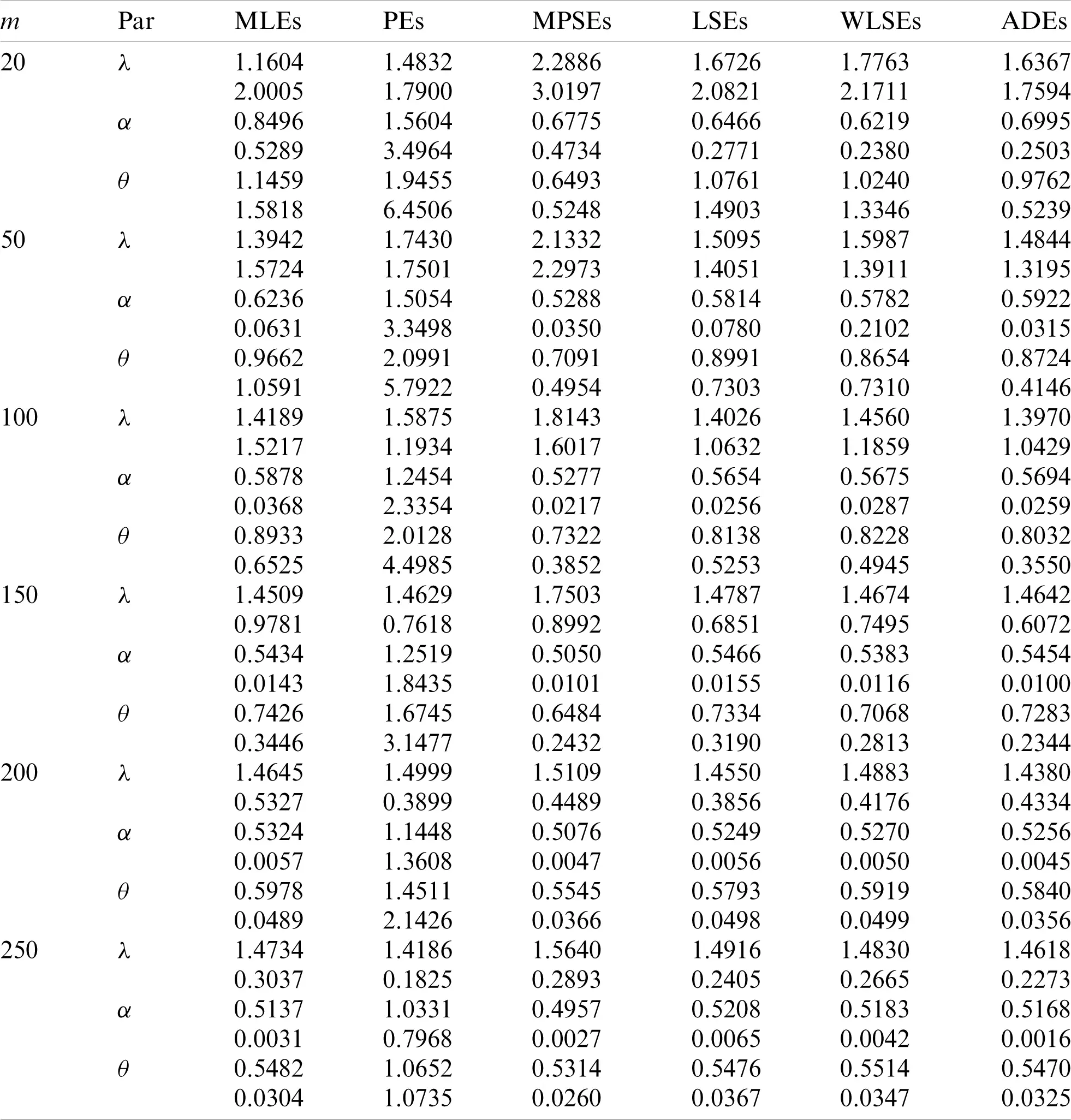Table 2: Estimates and MSEs for different estimates of λ=0.5 and α=θ=2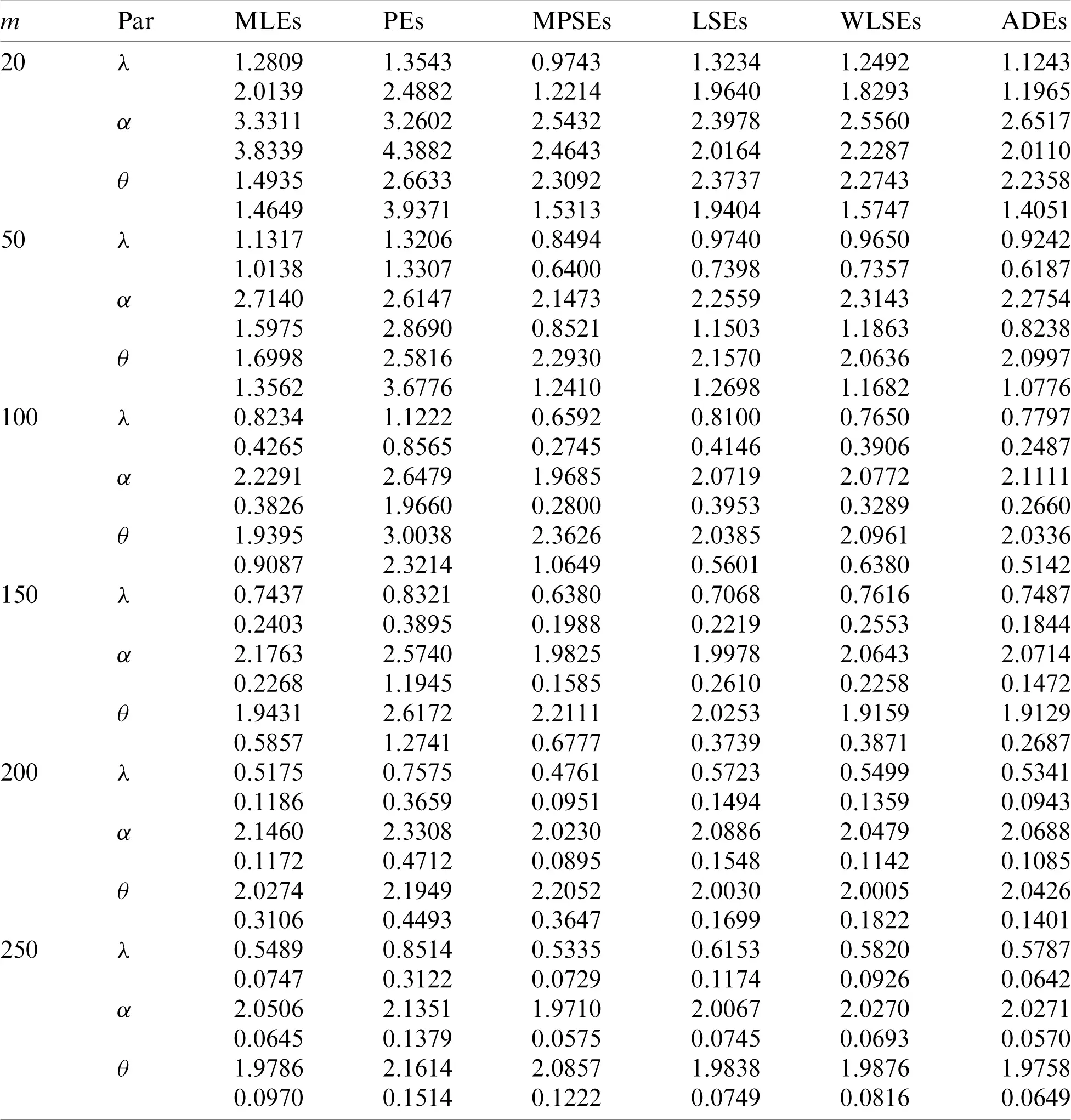6  Applications

To show the applicability of the proposed distribution and the different estimators derived in the previous sections two real data sets are analyzed. The first data set considered by  which represent life times (in days) of 39 liver cancers patients taken from Elminia cancer center Ministry of Health in Egypt. The second data set consists of 29 time between failures of a piece of construction equipment in chronological studied by . These data are displayed in Tab. 3.

Table 3: Real data sets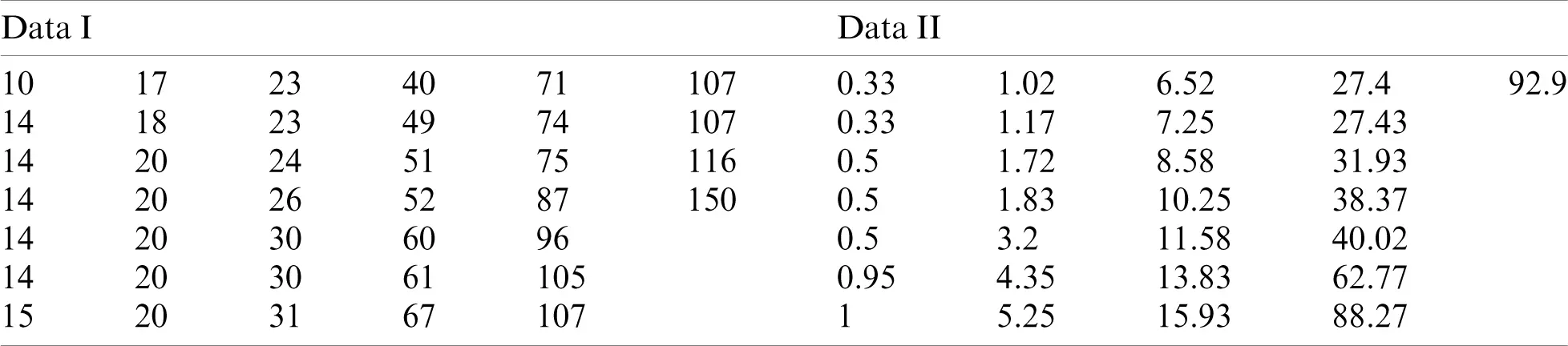We compare the results of the MLTIL distribution with IL distribution, inverse Weibull (IW) distribution, APIL distribution by  and alpha power inverse Weibull (APIW) distribution by . The density functions of these distributions (for x > 0) are as follow fIW(x;α,θ)=αθαx-α-1e-(θ/x)α, fAPIL(x;λ,α,θ)=log(λ)λ-1αθx-2(1+θ/x)-α-1λ(1+θ/x)-α, and fAPIW(x;λ,α,θ)=log(λ)λ-1αθx-α-1e-θx-αλe-θx-α.

We first obtain the MLEs of the competitive distributions. These estimates are presented in Tabs. 4 and 5 for datas 1 and 2, respectively. To compare the performance of the difference distributions to fit the data set, we use Kolmogorov-Smirnov (K-S) distance and the corresponding p-value. These statistics are also presented in Tabs. 4 and 5 for datas 1 and 2, respectively. From the results in these Tables it is observed that the MLTIL distribution has the smallest K-S distance with the highest p-value among other competitive distributions, therefore we conclude that the MLTIL distribution is the suitable model to fit these data. The fitted PDF, CDF, SF and P-P plot of the MLTIL distribution are presented in Figs. 3 and 4 for datas 1 and 2, respectively. These Figures support the results discussed before that the MLTIL distribution provides a close fit to these data sets.

Table 4: MLEs, K-S and p-value of real data 1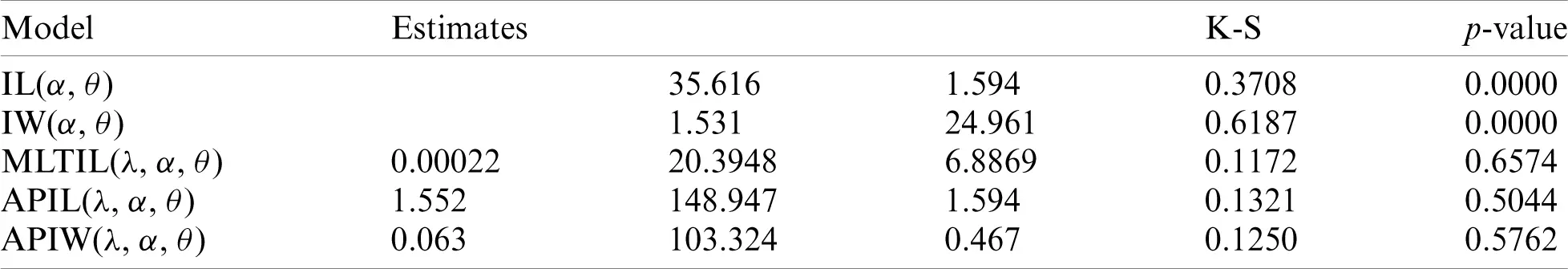Table 5: MLEs, K-S and p-value of real data 2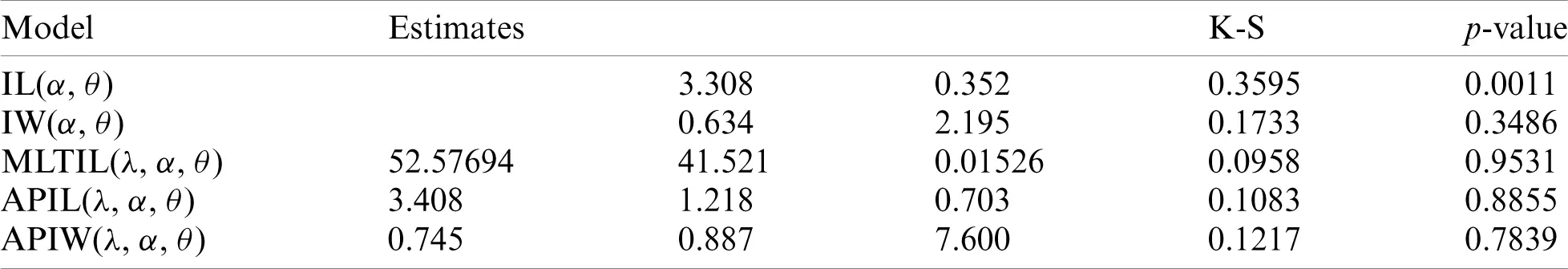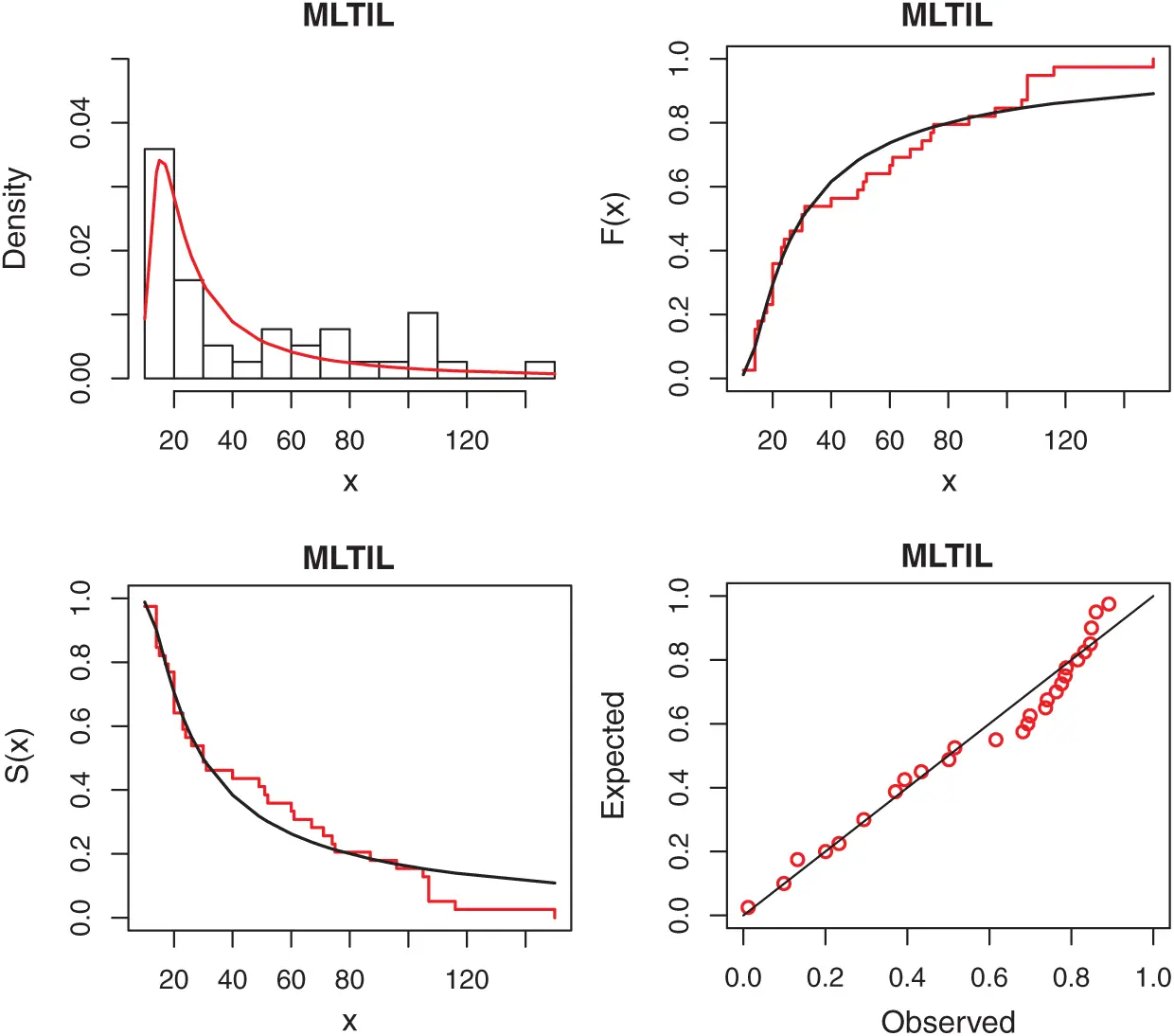Figure 3: Fitted density, estimated CDF and SF, and P-P plots for real data 1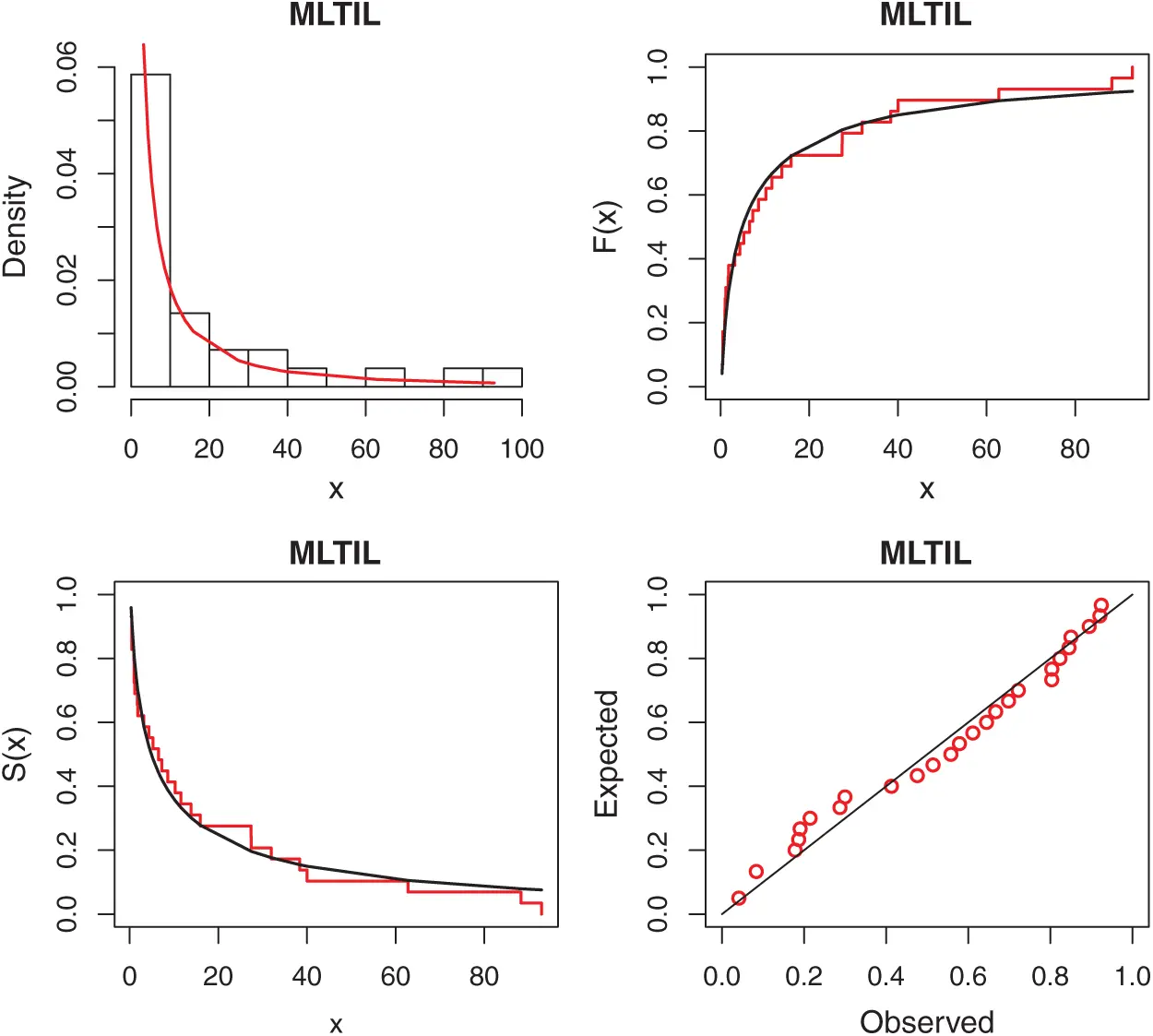Figure 4: Fitted density, estimated CDF and SF, and P-P plots for real data 2

To see which estimation method provide a good fit to these data we compare the other estimation methods with the maximum likelihood methods based on K-S distance and its p-value. For the two data sets, the various estimates, K-S distance and its p-value are obtained in Tabs. 6 and 7, respectively. Comparing the fitting of the different methods we can observe that the ADEs provides a good fit based on minimum K-S distance with highest p-value. Therefore, we can recommend to use the AD method to estimate the MLTIL distribution parameters from these real data sets. Also, the histogram and the fitted MLTIL distribution based on the different methods are presented in Fig. 5 for the two data sets.

Table 6: Various estimates, K-S and p-value of real data 1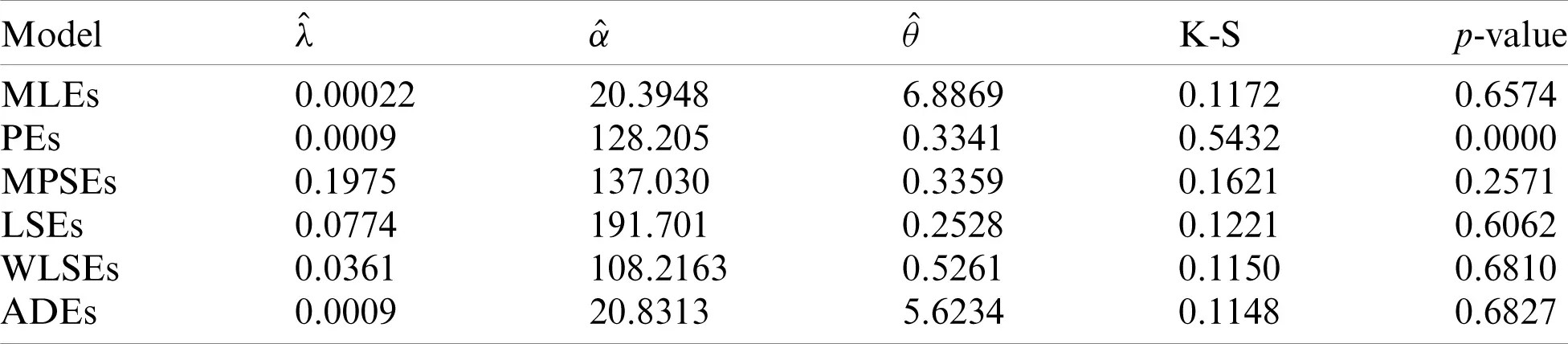Table 7: Various estimates, K-S and p-value of real data 2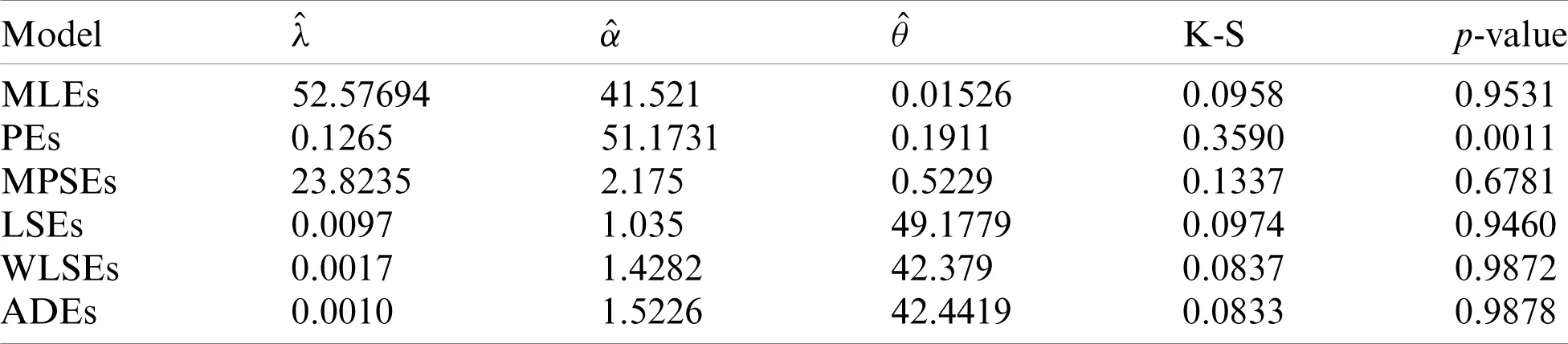Figure 5: Histogram and the fitted MLTIL density using different estimation methods for (a) data 1 and (b) data 2

7  Conclusion

In this paper, we have considered and studied a new generalization of the traditional inverse Lomax distribution by adding a new shape parameter. We have used the logarithmic transformed method for this purpose and a new three parameters inverse Lomax distribution which called modified logarithmic transformed inverse Lomax distribution is introduced. The new distribution has different failure rate shapes, so it can be used in analyzing lifetime data. Some statistical properties of the new distribution are derived including quantiles, moments, probability weighted moments, entropies, residual life, stress-strength parameter and order statistics. To estimate the parameters of the proposed distribution, six classical methods are considered. To compare the efficiency of these methods a simulation study is performed and the performance of the different estimators is compared. To show the applicability of the new distribution, two real data sets are analyzed which indicate that our new distribution perform better than some other competitive distributions. Also, the numerical illustration revealed that the Anderson Darling estimation method is the best method to estimate the proposed distribution parameters. In this study, the proposed distribution shows its ability in modelling engineering and medical data sets where traditional and some recently proposed models cannot be used for this purpose. We hope that this model attract wider sets of applications in the other different fields.

Acknowledgement: The author would like to thank the editor and the two reviewers for their constructive comments which improve the quality of the paper.

Funding Statement: This project was funded by the Deanship Scientific Research (DSR), King Abdulaziz University, Jeddah under Grant No. (RG-14-130-41). The author, therefore, acknowledge with thanks DSR for technical and financial support.

Conflict of Interest: The authors declare that they have no conflicts of interest to report regarding the present study.

## References

1. Louzada, F., Ramos, P. L., & Nascimento, D. (2018). The inverse nakagami-m distribution: A novel approach in reliability. IEEE Transactions on Reliability, 67(3), 1030-1042. [Google Scholar] [CrossRef]
2. Eliwa, M. S., El-Morshedy, M., & Ibrahim, M. (2019). Inverse Gompertz distribution: Properties and different estimation methods with application to complete and censored data. Annals of Data Science, 6(2), 321-339. [Google Scholar] [CrossRef]
3. Ramos, P. L., Louzada, F., Shimizu, T. K., & Luiz, A. O. (2019). The inverse weighted Lindley distribution: Properties, estimation and an application on a failure time data. Communications in Statistics-Theory and Methods, 48(10), 2372-2389. [Google Scholar] [CrossRef]
4. Hassan, A. S., & Abd-Allah, M. (2019). On the inverse power Lomax distribution. Annals of Data Science, 6(2), 259-278. [Google Scholar] [CrossRef]
5. Hassan, A. S., & Mohamed, R. E. (2019). Weibull inverse lomax distribution. Pakistan Journal of Statistics and Operation Research, 15(3), 587-603. [Google Scholar] [CrossRef]
6. Maxwell, O., Chukwu, A. U., Oyamakin, O. S., & Khaleel, M. (2019). The Marshall–Olkin inverse lomax distribution (MO-ILD) with application on cancer stem cell. Journal of Advances in Mathematics and Computer Science, 33, 1-12. [Google Scholar] [CrossRef]
7. ZeinEldin, R. A., Haq, M. A., Hashmi, S., & Elsehety, M. (2020). Alpha power trans- formed inverse lomax distribution with different methods of estimation and applications. Complexity, 2020(1), 1-15. [Google Scholar] [CrossRef]
8. Pappas, V., Adamidis, K., Loukas, S. (2012). A family of lifetime distributions. International Journal of Quality, Statistics, and Reliability, 1–6. DOI 10.1155/2012/760687. [CrossRef]
9. Al-Zahrani, B., Marinho, P. R. D., Fattah, A. A., Ahmed, A. H. N., & Cordeiro, G. M. (2016). The (P-A-L) extended Weibull distribution: A new generalization of the Weibull distribution. Hacettepe Journal of Mathematics and Statistics, 45, 851-868. [Google Scholar]
10. Dey, S., Nassar, M., & Kumar, D. (2017). Alpha logarithmic transformed family of distributions with applications. Annals of Data Science, 4(4), 457-482. [Google Scholar] [CrossRef]
11. Eltehiwy, M. (2018). Logarithmic inverse Lindley distribution: Model, properties and applications. Journal of King Saud University–Science, 32(1), 136-144. [Google Scholar] [CrossRef]
12. Dey, S., Nassar, M., Kumar, D., & Alaboud, F. (2019). Alpha logarithm transformed fréchet distribution: Properties and estimation. Austrian Journal of Statistics, 48(1), 70-93. [Google Scholar] [CrossRef]
13. Fisher, B., & Kilicman, A. (2012). Some results on the gamma function for negative integers. Applied Mathematics Information Sciences, 6, 173-176. [Google Scholar]
14. Gradshteyn, I. S., Ryzhik, I. M. (2014). Table of integrals, series, and products. sixth edition. San Diego: Academic Press.
15. Akgul, A. (2020). Analysis and new applications of fractal fractional differential equations with power law kernel. Discrete Continuous Dynamical Systems-S, DOI 10.3934/dcdss.2020423. [CrossRef]
16. Atangana, A., Akgul, A., & Owolab, K. M. (2020). Analysis of fractal fractional differential equations. Alexandria Engineering Journal, 59(3), 1117-1134. [Google Scholar] [CrossRef]
17. Owolab, K. M., Atangana, A., & Akgul, A. (2020). Modelling and analysis of fractal-fractional partial differential equations: Application to reaction-diffusion model. Alexandria Engineering Journal, 59(4), 2477-2490. [Google Scholar] [CrossRef]
18. Kao, J. H. (1958). Computer methods for estimating Weibull parameters in reliability studies. IRE Transactions on Reliability and Quality Control, 13, 15-22. [Google Scholar] [CrossRef]
19. Kao, J. H. K. (1958). A graphical estimation of mixed Weibull parameters in life testing electron tube. Technometrics, 1(4), 389-407. [Google Scholar] [CrossRef]
20. Santo, A. P. J., & Mazucheli, J. (2014). Comparison of estimation methods for the Marshall–Olkin extended Lindley distribution. Journal of Statistical Computation and Simulation, 85(17), 3437-3450. [Google Scholar] [CrossRef]
21. Nassar, M., Afify, A. Z., Dey, S., & Kumar, D. (2018). A new extension of Weibull distribution: Properties and different methods of estimation. Journal of Computational and Applied Mathematics, 336(1), 439-457. [Google Scholar] [CrossRef]
22. Cheng, R. C. H., Amin, N. A. K. (1979). Maximum product of spacings estimation with applications to the lognormal distribution. Math Report, 791.
23. Cheng, R. C. H., & Amin, N. A. K. (1983). Estimating parameters in continuous univariate distributions with a shifted origin. Journal of the Royal Statistical Society: Series B (Methodological), 45(1983), 394-403. [Google Scholar]
24. Swain, J., Venkatraman, S., & Wilson, J. (1988). Least squares estimation of distribution function in Johnsons translation system. Journal of Statistical Computation and Simulation, 29(4), 271-297. [Google Scholar] [CrossRef]
25. Mahmoud, M. A. W., Attia, F. A., Taib, I. B. (2005). Test for exponential better than used in average based on the total time on test transform. The Proceeding of the 17th Annual Conference on Statistics, Modelling in Human and Social Science, pp. 29–30. Cairo, Egypt.
26. Fan, Q., & Fan, H. Q. (2015). Reliability analysis and failure prediction of construction equipment with time series models. Journal of Advanced Management Science, 3, 203-210. [Google Scholar] [CrossRef]
27. Basheer, A. M. (2019). Alpha power inverse Weibull distribution with reliability application. Journal of Taibah University for Science, 13(1), 423-432. [Google Scholar] [CrossRef]This work is licensed under a Creative Commons Attribution 4.0 International License, which permits unrestricted use, distribution, and reproduction in any medium, provided the original work is properly cited.